# 1. Refer to the table which summarizes the results of testing for a certain disease. Positive...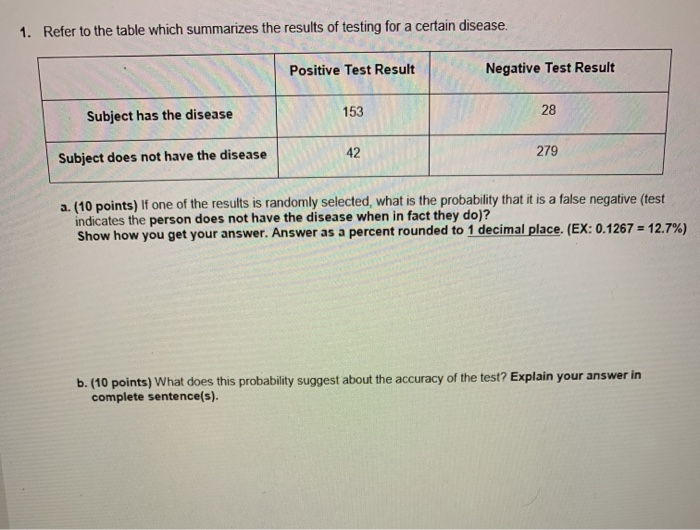1. Refer to the table which summarizes the results of testing for a certain disease. Positive Test Result Negative Test Result 153 28 Subject has the disease 42 279 Subject does not have the disease a. (10 points) If one of the results is randomly selected, what is the probability that it is a false negative (test indicates the person does not have the disease when in fact they do)? Show how you get your answer. Answer as a percent rounded to 1 decimal place. (EX: 0.1267 = 12.7%) b. (10 points) What does this probability suggest about the accuracy of the test? Explain your answer in complete sentence(s).

1.

According to the question,

 Positive Test Result Negative Test Result Total Subject has the disease 153 28 181 Subject does not have the disease 42 279 321 Total 195 307 502

a.

Probability of false negative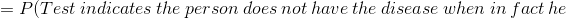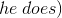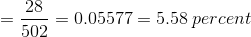b.

As the probability of false negative is as low as 5.58% we can conclude that the test is about 95% accurate. In other words, in a random sample of 100 approximately in 95 cases the test will rightly diagnose the disease.

##### Add Answer of: 1. Refer to the table which summarizes the results of testing for a certain disease. Positive...
Similar Homework Help Questions
• ### 1. Refer to the table which summarizes the results of testing for a certain disease. Positive...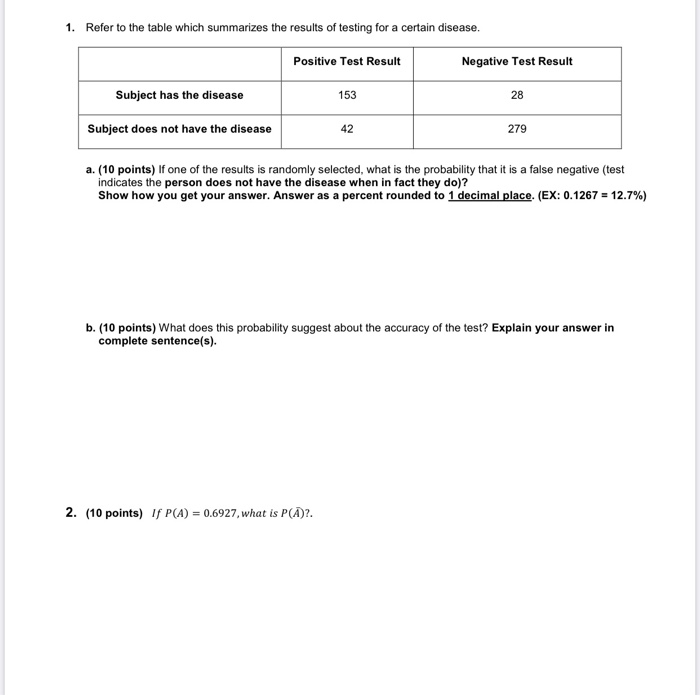1. Refer to the table which summarizes the results of testing for a certain disease. Positive Test Result Negative Test Result Subject has the disease 153 28 Subject does not have the disease 42 279 a. (10 points) If one of the results is randomly selected, what is the probability that it is a false negative (test indicates the person does not have the disease when in fact they do)? Show how you get your answer. Answer as a percent...

• ### 1. Find the indicated probability (1 point) Refer to the table which summarizes the results of...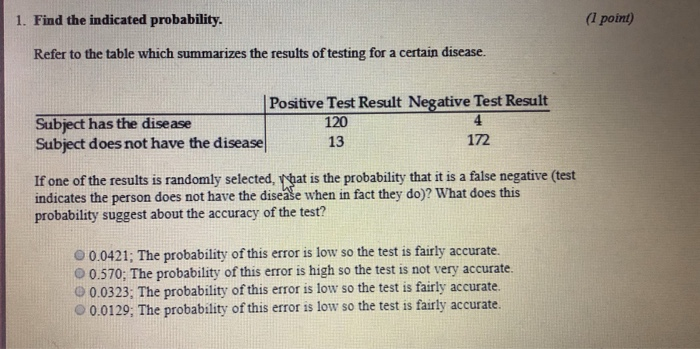1. Find the indicated probability (1 point) Refer to the table which summarizes the results of testing for a certain disease. Positive Test Result Negative Test Result Subject has the disease Subject does not have the disease 120 13 172 If one of the results is randomly selected, what is the probability that it is a false negative (test indicates the person does not have the disease when in fact they do)? What does this probability suggest about the accuracy...

• ### The individual actually had the disease Yes No The accompanying table shows the results from a...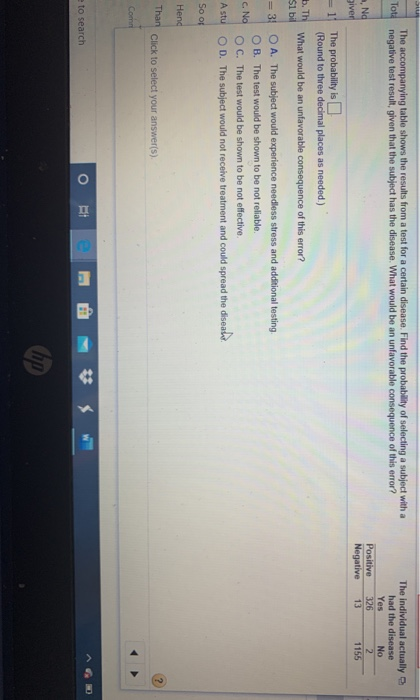The individual actually had the disease Yes No The accompanying table shows the results from a test for a certain disease. Find the probability of selecting a subject with a negative test result, given that the subject has the disease. What would be an unfavorable consequence of this error? ott Positive Negative 13 1155 Nd The probability is (Round to three decimal places as needed.) TH What would be an unfavorable consequence of this error? 51 bi O A. The...

• ### The table below shows the predicted testing outcome for a disease that affects 2% of a...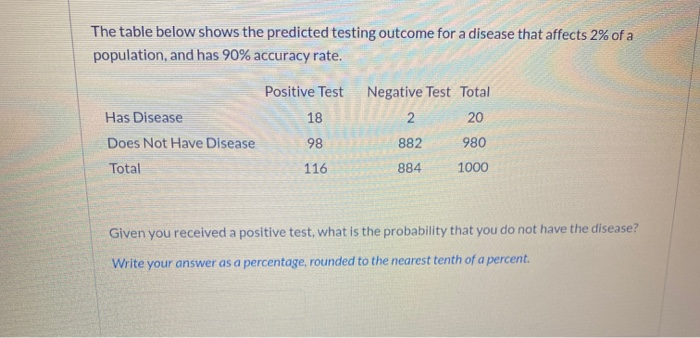The table below shows the predicted testing outcome for a disease that affects 2% of a population, and has 90% accuracy rate. Positive Test Negative Test Total Has Disease 18 2 20 Does Not Have Disease 98 882 980 Total 116 1000 884 Given you received a positive test, w he probability that you do not have the disease? Write your answer as a percentage, rounded to the nearest tenth of a percent.

• ### Question 24 4 pts The table below shows the predicted testing outcome for a disease that...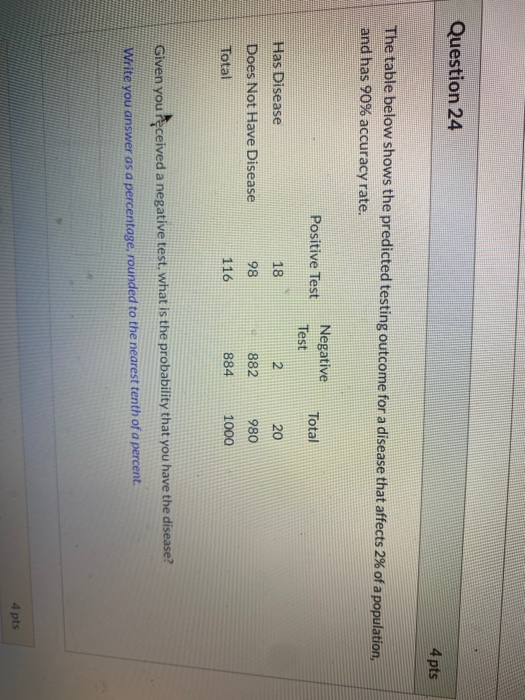Question 24 4 pts The table below shows the predicted testing outcome for a disease that affects 2% of a population, and has 90% accuracy rate. Positive Test Negative Test Total Has Disease 18 2 20 Does Not Have Disease 98 882 980 Total 116 884 1000 Given you received a negative test, what is the probability that you have the disease? Write you answer as a percentage, rounded to the nearest tenth of a percent. 4 pts

• ### 15. Use the following results from a test for marijuana​ use, which is provided by a...

15. Use the following results from a test for marijuana​ use, which is provided by a certain drug testing company. Among 142 subjects with positive test​ results, there are 28 false positive​ results; among 150 negative​ results, there are 3 false negative results. If one of the test subjects is randomly​ selected, find the probability of a false positive or false negative.​ (Hint: Construct a​ table.) What does the result suggest about the​test's accuracy? The probability of a false positive...

• ### I. You are just told by your doctor that you tested positive for a serious disease....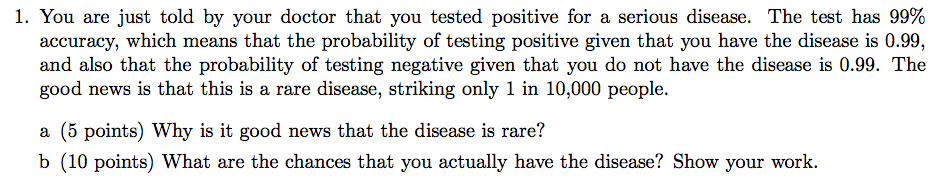I. You are just told by your doctor that you tested positive for a serious disease. The test has 99% accuracy, which means that the probability of testing positive given that you have the disease is 0.99, and also that the probability of testing negative given that you do not have the disease is 0.99. The good news is that this is a rare disease, striking only 1 in 10,000 people. a (5 points) Why is it good news that...

• ### Only 1 in 1,000 is afflicted with a rare disease for which a diagnostic test has...

Only 1 in 1,000 is afflicted with a rare disease for which a diagnostic test has been developed. When a person has the disease , the test returns a positive result 99% of the time. However, when a person does not have the disease, the test shows a positive result only 2% of the time. When a person's test results are positive, in order to validate the results, a second test is given. The second test has the same accuracy...

• ### Negative Test Result Positive Test Result 335 Hepatitis C No Hepatitis C DIR (a) (2 points)...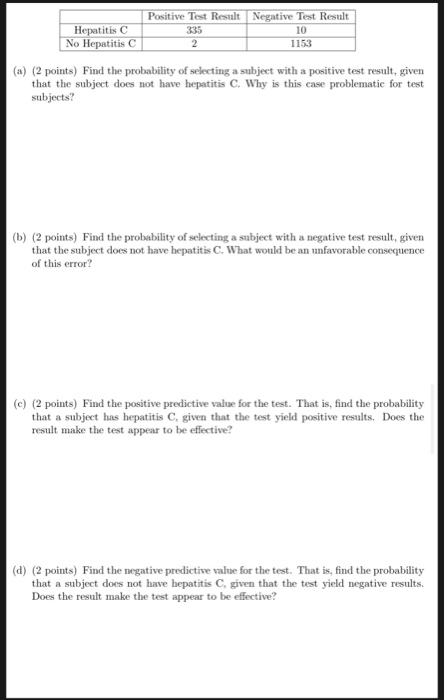Negative Test Result Positive Test Result 335 Hepatitis C No Hepatitis C DIR (a) (2 points) Find the probability of selecting a subject with a positive test result, given that the subject does not have hepatitis C Why is this case problematic for test subjects? (b) (2 points) Find the probability of selecting a subject with a negative test result, given that the subject does not have hepatitis C. What would be an unfavorable consequence of this error? (c) (2...

• ### #22 is needed Professor's statement -  you have to first construct the table these results from the "1-Panel-TH...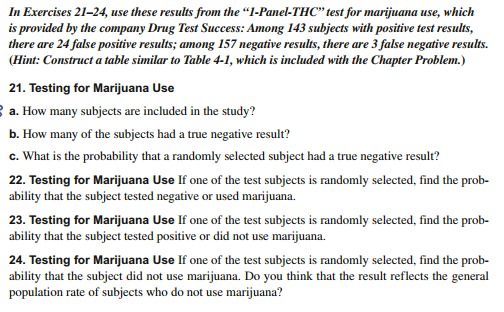#22 is needed Professor's statement -  you have to first construct the table these results from the "1-Panel-THC" test for marijuana use, which is provided by the company Drug Test Success: Among 143 subjects with positive test results, there are 24 false positive results; among 157 negative results, there are 3 false negative results. (Hint: Construct a table similar to Table 4-1, which is included with the Chapter Problem.) In Exercises 21-24, 1 use 21. Testing for Marijuana Use a....

Free Homework App